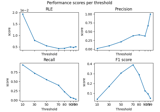# mne.simulation.metrics.region_localization_error#

mne.simulation.metrics.region_localization_error(stc_true, stc_est, src, threshold='90%', per_sample=True)[source]#

Compute region localization error (RLE) between 2 source estimates.

$RLE = \frac{1}{2Q}\sum_{k \in I} \min_{l \in \hat{I}}{||r_k - r_l||} + \frac{1}{2\hat{Q}}\sum_{l \in \hat{I}} \min_{k \in I}{||r_k - r_l||}$

where $$I$$ and $$\hat{I}$$ denote respectively the original and estimated indexes of active sources, $$Q$$ and $$\hat{Q}$$ are the numbers of original and estimated active sources. $$r_k$$ denotes the position of the k-th source dipole in space and $$||\cdot||$$ is an Euclidean norm in $$\mathbb{R}^3$$.

Parameters:
stc_trueinstance of (Vol|Mixed)SourceEstimate

The source estimates containing correct values.

stc_estinstance of (Vol|Mixed)SourceEstimate

The source estimates containing estimated values e.g. obtained with a source imaging method.

srcinstance of SourceSpaces

The source space on which the source estimates are defined.

threshold

The threshold to apply to source estimates before computing the dipole localization error. If a string the threshold is a percentage and it should end with the percent character.

per_samplebool

If True the metric is computed for each sample separately. If False, the metric is spatio-temporal.

Returns:
metricfloat | array, shape (n_times,)

The metric. float if per_sample is False, else array with the values computed for each time point.

Notes

Papers  and  use term Dipole Localization Error (DLE) for the same formula. Paper  uses term Error Distance (ED) for the same formula. To unify the terminology and to avoid confusion with other cases of using term DLE but for different metric , we use term Region Localization Error (RLE).

New in v1.2.

References

## Examples using mne.simulation.metrics.region_localization_error#Compare simulated and estimated source activity

Compare simulated and estimated source activity